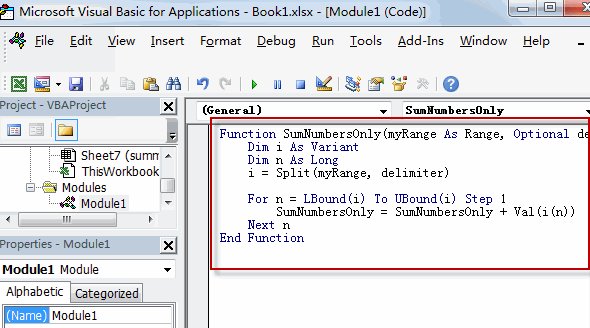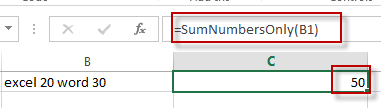# Excel Sum Numbers Ignore Text

This post will guide you how to sum numbers ignore text in same cell in Excel. How do I sum numbers with text appended in the same cell with a formula in excel.How to sum only numbers in a cells with text and numbers using User Defined Function in Excel.

## Sum Numbers Ignore Text

To sum numbers only in the same cell with text and numbers in Excel, you can write an User Defined function with VBA code in Excel. Just do the following steps:

#1 open your excel workbook and then click on “Visual Basic” command under DEVELOPER Tab, or just press “ALT+F11” shortcut.#2 then the “Visual Basic Editor” window will appear.

#3 click “Insert” ->”Module” to create a new module.#4 paste the below VBA code into the code window. Then clicking “Save” button.```Function SumNumbersOnly(myRange As Range, Optional delimiter As String = " ") As Double
Dim i As Variant
Dim n As Long
i = Split(myRange, delimiter)

For n = LBound(i) To UBound(i) Step 1
SumNumbersOnly = SumNumbersOnly + Val(i(n))
Next n
End Function```

#5 back to the current worksheet, try to enter the below formula in Cell C1.

`=SumNumbersOnly(B1)`